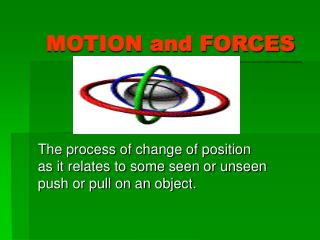# MOTION and FORCES - PowerPoint PPT PresentationDownload PresentationMOTION and FORCES

MOTION and FORCES
Download Presentation## MOTION and FORCES

- - - - - - - - - - - - - - - - - - - - - - - - - - - E N D - - - - - - - - - - - - - - - - - - - - - - - - - - -
##### Presentation Transcript

1. MOTION and FORCES The process of change of position as it relates to some seen or unseen push or pull on an object.

2. Newton’s Three (3) Laws of Motion • INERTIA • ACCELERATION • REACTIONARY FORCES

3. INERTIA • An object at rest will remain at rest or an object in motion will remain in motion unless acted upon by some outside force. Example: Riding in a car and then suddenly apply the brakes; your body continues to move forward.

4. ACCELERATION • An object of a certain size and MASS will accelerate depending on the size of the FORCE acting upon it and the DIRECTION in which it acts. Example: A TRACTOR TRAILER TRUCK and a VOLKSWAGON CAR with a DEISEL ENGINE and 4 CYLINDER ENGINE going UPHILL or DOWNHILL.

5. REACTIONARY FORCES • For every Action there is an equal and opposite Reaction. Example: Fill a balloon with air, let the balloon go and allow for the air to escape to observe the balloon’s movement.

6. Definitions • Speed- how fast an object moves. • Velocity- how fast an object moves, with specific reference to DIRECTION. • Acceleration- how Speed/Velocity changes with time (a change in speed). • Force- any push or pull on an object. • Momentum- an objects mass multiplied by it’s Velocity.

7. FORMULAS Speed = Distance/Time S=D/T Acceleration=Velocity(final)-Velocity(initial) Time Momentum = Mass X Velocity Force = Mass X Acceleration

8. Units of Motion • SPEED – m/s, mph, km/hr etc. • ACCELERATION – m/s² or km/hr/sec Constant for acceleration due to GRAVITY. g = 9.8 m/s² • MOMENTUM – kg x m/s

9. Sample Motion Problems • Calculate the speed of a runner that ran 55m in 5.5sec. ___________________ • What is the velocity of a car that traveled 150km N in 3hrs? __________ • How fast does a Porsche accelerate from a standstill in 10sec to a top speed of 120km/hr?_____________________ USE THE TRIANGLE TO WORK PROBLEMS!!!

11. VECTORS • Vectors is a way of representing the magnitude and direction of velocities, forces, etc. with the use of arrows. • Resultant Vector is found when you either use the Pythagorean Theorem for vectors at right angles to each other or use graphical addition.

12. PYTHAGOREAN THEOREM • In order to find the length of a resultant vector where two vectors are at right angles to one another you must use the following formula: a² + b² = c² (See sample problems worked by teacher)

13. Graphical Addition • Another method for addition of vectors is to manipulate their graphical representations on paper. A ruler is needed to draw the vectors to the correct length. A protractor is needed to insure the correct angles. The length of the arrows should be proportional to the magnitude of the quantity represented.

14. Rules to Remember • When drawing vectors, always use a straight edge. • Vectors should always be TIP to TAIL. • All vector lengths should be proportional to the magnitude of the quantity. • BE ACCURATE, ACCURATE, ACCURATE!

15. FORCE • A FORCE is any push or pull on an object. • BALANCED FORCES occur when two or more forces act in different directions on an object and the NET FORCE is ZERO. • UNBALANCED FORCES occur when two or more forces act in different directions on an object and a NET FORCE occurs in the direction of the larger force.

16. TYPES of FORCES • FRICTIONAL FORCE- is the resistance of motion of an object. • GRAVITATIONAL FORCE- is the attraction between two objects that is determined by the size and distance of the objects. • CENTRIPETAL FORCE- the force exerted on an object in a circular motion.

17. FORCE FORMULA • Force = Mass X Acceleration F = ma Unit of Force is the NEWTON. This is an abbreviation for kg x m/s². Acceleration of a falling object due to GRAVITY is called g. The constant for g = 9.8m/s²

18. Sample Problem • What is the force of a 250 kg linebacker that hits a dummy at an acceleration of 5 m/s² ? To solve: F=? F=ma = (250kg)(5m/s²) M=250kg = 1250N A=5m/s²

19. Universal Law of Gravitation • Every object in the Universe pulls on every other object. • The more mass an object has, the greater it’s gravitational force (pull). • The greater the distance between two objects, the less attraction they have for each other.

20. Inverse Square Law • This is the relationship between gravitational force proportional to the inverse square of the distance between the objects. Simply put, the Gravitational Force increases with increased Mass and decreases as the distance between the masses becomes greater.

21. Weight vs Mass • Gravity gives the MASS of an object it’s WEIGHT. • Mass measures the amount of matter in an object. • Weight is a force that changes depending on the amount of gravity pulling on an object.